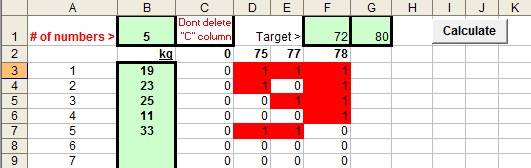Handy Excel Macros
(Microsoft Excel 2003)

This will give combinations of numbers that sum up to between the two target values.

Enter numbers into the light blue/green areas on the sheet.  The sum of the number combinations is then generated.

You get a range of choices between the two target values you specify.

Target  - enter the two number values where you want your numbers to sum between those values eg if you enter 72 & 80

the program finds possible combinations adding up to between 72 and 80.  (So one result 75 is made up of 19 + 23 + 33)

# of numbers = how many numbers there are.   Note: as you add more numbers  the calculations involved increase exponentially.

30 numbers seems to be the limit for my computer to calculate an answer from. - (a modern computer).

If your computer says its not responding but it looks as if its calculating then it may be OK but it may take a very long time to calculate

all the answers as you have entered too many numbers.  Press escape to exit the macro.

Do not manually delete the previous results when you want to recalculate.  The program will delete the old

results as it recalculates the new values you enter.  Remember to change the number of numbers value if needed.

.

Sum to target

Screenshot:(includes PayPal) -
- you choose the amount)